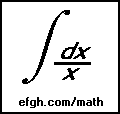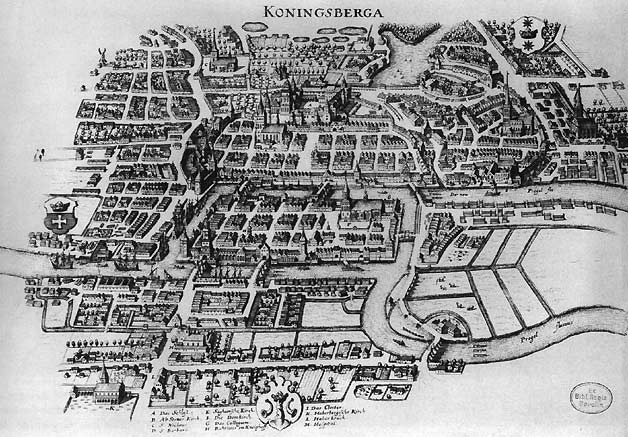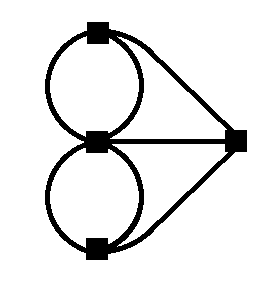# The Bridges of Königsberg

#### June 9, 2015

One of the classic problems in mathematics is the Bridges of Königsberg, which was solved by the Swiss mathematician Leonhard Euler in the eighteenth century. It is easy to state and fairly easy to solve.

A river passes through the city of Königsberg (now Kalingrad). In the river are two islands. Seven bridges connected the islands and the banks, as shown on this old map:The two river channels come together somewhere off the right side of the map.

Many people tried to walk around Königsberg, crossing each bridge once and only once, but nobody could do it. Euler showed that the task is impossible. His proof requires only some basic knowledge of arithmetic and the properties of even and odd numbers.

Euler's brilliant insight, if you can call it that, was to count the number of bridge ENDS connected to each piece of land:

• 3 on the north bank
• 3 on the south bank
• 5 on the island in the center of the map
• 3 on the island at the right side of the map

A walk that crosses each bridge once and only once is called, suitably enough, an Euler walk (or an Euler path).

If an Euler walk does not begin or end at a particular piece of land, then there must be an EVEN NUMBER of bridges connected to it, because an Euler walker who enters the area by one bridge must leave it by another. If an Euler walk begins or ends at a particular piece of land, then the number of bridges connected to it must be ODD.

Since every one of the four pieces of land has an odd number of bridges connected to it, it is obvious than an Euler walk is impossible.

The Bridges of Königsberg is an example of a general mathematical structure called a graph.

A graph consists of a finite number of vertices (also called nodes), corresponding to the pieces of land in the Bridges of Königsberg, and a finite number of edges (also called lines), corresponding to the bridges. We will continue to call them bridges. Also, to avoid difficulties with nonexistent walks, we require that there must be at least one vertex.

Here is a more abstract representation of the Königsberg graph:A graph can be more general than the Bridges of Königsberg. For example, a bridge may connect a vertex to itself, and the vertices need not be confined to a single plane. An isolated vertex, with no connecting bridges, is also possible.

The number of bridges connecting a particular vertex is called its degree (or valency).

Euler's arguments showed that an Euler walk is possible only if either (1) all vertices are of even degree, in which case the walk starts and ends at the same vertex, or (2) two vertices, where the walk begins and ends, are of odd degree and all other vertices are of even degree.

Two properties of Euler walks are obvious. The reverse of an Euler walk is an Euler walk, and if an Euler walk begins and ends at the same vertex, it can also be begun and ended at any other vertex.

Are these conditions also sufficient? Obviously not. The graph must also be connected, that is, there must be some path (not necessarily an Euler path) from every vertex to every other vertex.

Proving the existence of an Euler walk for a connected graph with no vertices of odd degree or two vertices of odd degree is somewhat more difficult, but it involves no advanced mathematics.

First, consider a connected graph where all vertices are of even degree. Start walking at one vertex, keeping track of which bridges you have crossed. Every time you enter a vertex, leave it by a bridge you haven't crossed previously. If it is not the starting vertex, then if you entered by one uncrossed bridge, you can always leave it by another. And after you leave, the vertex still has an even number of uncrossed bridges.

The walk must come to an end when you return to the starting vertex and can't find an uncrossed bridge to leave it.

If you have managed to cross every bridge, the walk is finished.

If there is still at least one uncrossed bridge, the walk must be augmented.

Notice, however, that every vertex still has an even number of uncrossed bridges.

Since the graph is connected, there is a path from the starting vertex to a vertex with an uncrossed bridge. Consider the first vertex in this path with an uncrossed bridge. It must be in the walk. Call it vertex A.

Now start at vertex A and do a second walk, crossing only bridges that you haven't already crossed, either in this walk or the first one. As previously, the walk ends only when you return to vertex A.

Now combine the two walks into a single walk. The easiest way to do this is to start first walk at vertex A. When you come back to vertex A, do the second walk.

If there is still at least one uncrossed bridge, you can continue this process until all bridges have been crossed.

You might ask what happens if the graph has only one vertex and at least one bridge. It is easy to show that the graph is connected, the vertex has even degree, and there is an Euler walk. A graph with only one vertex and no bridges is not necessarily an exception, although the concepts of connectedness and Euler walks are somewhat vacuous in this case.

This is the desired result for a connected graph in which every vertex is of even degree. Now consider a graph with two vertices B and C of odd degree. Create a slightly larger graph by inserting a bridge connecting vertices B and C. All vertices in this graph are of even degree, so it has an Euler walk. One part of this walk involves crossing the new bridge from vertex B to vertex C, or vice-versa.

It is clear that this Euler walk, without the new crossing between vertices B and C, is an Euler walk on the original graph.

An Uzbek translation of this page has been posted at eduworksdb.com/the-bridges-of-konigsberg by Sherali Niyazova.